Study Materials: ncert solutions

Our ncert solutions for Exercise 6.2 Class 9 maths 6. Lines and Angles - ncert solutions - Toppers Study is the best material for English Medium students cbse board and other state boards students.

Solutions ⇒ Class 9th ⇒ Mathematics ⇒ 6. Lines and Angles

# Exercise 6.2 Class 9 maths 6. Lines and Angles - ncert solutions - Toppers Study

Topper Study classes prepares ncert solutions on practical base problems and comes out with the best result that helps the students and teachers as well as tutors and so many ecademic coaching classes that they need in practical life. Our ncert solutions for Exercise 6.2 Class 9 maths 6. Lines and Angles - ncert solutions - Toppers Study is the best material for English Medium students cbse board and other state boards students.

## Exercise 6.2 Class 9 maths 6. Lines and Angles - ncert solutions - Toppers Study

CBSE board students who preparing for class 9 ncert solutions maths and Mathematics solved exercise chapter 6. Lines and Angles available and this helps in upcoming exams 2023-2024.

### You can Find Mathematics solution Class 9 Chapter 6. Lines and Angles

• All Chapter review quick revision notes for chapter 6. Lines and Angles Class 9
• NCERT Solutions And Textual questions Answers Class 9 Mathematics
• Extra NCERT Book questions Answers Class 9 Mathematics
• Importatnt key points with additional Assignment and questions bank solved.

NCERT Solutions do not only help you to cover your syllabus but also will give to textual support in exams 2023-2024 to complete exercise 6.2 maths class 9 chapter 6 in english medium. So revise and practice these all cbse study materials like class 9 maths chapter 6.2 in english ncert book. Also ensure to repractice all syllabus within time or before board exams for ncert class 9 maths ex 6.2 in english.

See all solutions for class 9 maths chapter 6 exercise 6 in english medium solved questions with answers.

### Exercise 6.2 class 9 Mathematics Chapter 6. Lines and Angles

• Exercise 6.2 Class 9 Maths 6. Lines And Angles - Ncert Solutions - Toppers Study
• Class 9 Ncert Solutions
• Solution Chapter 6. Lines And Angles Class 9
• Solutions Class 9
• Chapter 6. Lines And Angles Exercise 6.2 Class 9

## Exercise 6.2 Class 9 maths 6. Lines and Angles - ncert solutions - Toppers Study

Chapter 6. Lines and Angles

Exercise 6.2

Q1. In Fig. 6.28, find the values of x and y and then show that AB || CD.

Solution: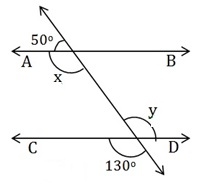x + 50° = 180° (linear pair)

x = 180° - 50°

x = 130°       ............. (1)

y = 130° (Vertically oposite angle) ....... (2)

From equation (1) and (2)

x = y = 130° (Alternate Interior Angle )

∴ AB || CD

(If alternate interior angle is equal then a pair of lines are parallel.)

Q2. In Fig. 6.29, if AB || CD, CD || EF and y : z = 3 : 7, find x.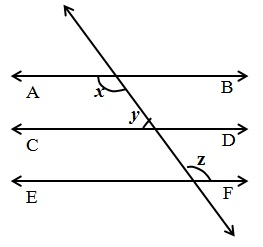Solution:

AB || CD  (Given) ............. (1)

CD || EF  (Given) ............. (2)

From equation (1) and (2)

AB || EF

∴ x = z  .......... (3) (Alternate Interior Angle)

y : z = 3 : 7 (Given)

Let y = 3k, z = 7k

∴ x = z = 7k   From equ. (3)

AB || CD  (Given)

Now, x + y = 180°  (Sum of interior adjacent angle is 180°)

⇒ 7k + 3k = 180°

⇒ 10k = 180°

⇒ k = 18°

x = 7k

= 7 × 18°

x = 126°

Q3. In Fig. 6.30, if AB || CD, EF ⊥ CD and ∠ GED = 126°, find ∠ AGE, ∠ GEF and ∠ FGE.​Solution:

∠GED = 126°

AB || CD and GE is a transversal.  (Given)

∴ ​ ∠AGE = ∠GED  (Alternate Interior Angle)

∴ ​ ∠AGE = 126°

EF ⊥ CD (Given)

∴ ∠FED = 90°  ............ (1)

Now, ∠GED = 126°

Or,     ∠GEF + ∠FED = 126°

∠GEF +  90°  = 126°      From eqa (1)

⇒       ∠GEF = 126° - 90°

⇒       ∠GEF = 36°

∠AGE + ∠FGE = 180°  (linear pair)

⇒  126° + ∠FGE = 180°

⇒  ∠FGE = 180° - 126°

⇒  ∠FGE = 154°

∠AGE = 126°, ∠GEF = 36°and ∠FGE = 154°

Q4. In Fig. 6.31, if PQ || ST, ∠ PQR = 110° and ∠ RST = 130°, find ∠ QRS.[Hint : Draw a line parallel to ST through point R.]

Solution:

Construction: Draw PQ || XY from point R.

∠ PQR = 110° and ∠ RST = 130°

PQ || ST  .............. (1) (Given)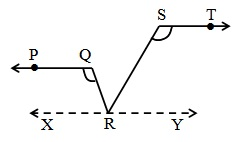PQ || XY .................(2) By construction.

From equa.(1) and (2) we get

ST || XY and SR is a transversal.

∴ ∠ RST + ∠ SRY = 180°

Or, 130° + ∠ SRY = 180°

⇒ ∠ SRY = 180° - 130°

⇒ ∠ SRY = 50°

PQ || XY and QR is a transversal

∴ ∠ PQR = ∠ QRY        (Alternate Interior Angle)

Or,  ∠ PQR = ∠ QRS +  ∠ SRY

⇒     110° = ∠ QRS + 50°

⇒     ∠ QRS = 110° - 50°

⇒     ∠ QRS = 60°

Q5. In Fig. 6.32, if AB || CD, ∠ APQ = 50° and ∠ PRD = 127°, find x and y.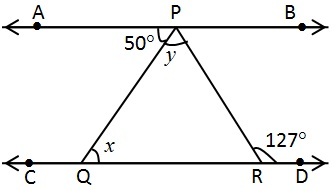Solution:

Given that:

∠ APQ = 50° and ∠ PRD = 127°

AB || CD and PQ is a transversal.

∴ ∠ PQR =  APQ  (Alternate Interior Angle)

Or, x = 50°

Similarily,

∠ APR = ∠ PRD  (Alternate Interior Angle)

50° + y = 127°

⇒ y = 127° - 50°

⇒ y = 77°

x = 50°, y = 77°

Q6. In Fig. 6.33, PQ and RS are two mirrors placed parallel to each other. An incident ray AB strikes the mirror PQ at B, the reflected ray moves along the path BC and strikes the mirror RS at C and again reflects back along CD. Prove that AB || CD.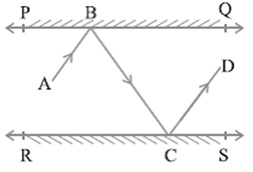Solution:

Given: PQ || RS and AB is incident ray, CD is reflected ray.

To prove: AB || CD

Construction:

Draw BM ⊥ PQ and CN ⊥ RS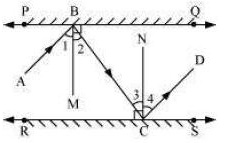Proof:

BM ⊥ PQ and CN ⊥ RS

∴ BM || CM and BC is a transverasal line

∴ ​∠2 = ∠ 3   ............ (1) (Alternate Interior Angle)

While we know that

Angle of incidence = Angle of reflection, where BM and CN are normal.

∴ ​∠1 = ∠ 2   .............. (2)

Similarily,

∴ ​∠3 = ∠ 4   .............. (3)

Using (1) (2) and (3) we get

∠1 = ∠ 4  ................ (4)

∠1 + ∠2 = ∠ 3 + ∠ 4

∠ABC = ∠ BCD  (Alternate Interior Angle)

Therefore, AB || CD proved

##### Other Pages of this Chapter: 6. Lines and Angles

Important Study materials for classes 06, 07, 08,09,10, 11 and 12. Like CBSE Notes, Notes for Science, Notes for maths, Notes for Social Science, Notes for Accountancy, Notes for Economics, Notes for political Science, Noes for History, Notes For Bussiness Study, Physical Educations, Sample Papers, Test Papers, Mock Test Papers, Support Materials and Books.Mathematics Class - 11th

NCERT Maths book for CBSE Students.

books

## Study Materials List:

##### Solutions ⇒ Class 9th ⇒ Mathematics
1. Number Systems
2. Polynomials
3. Coordinate Geometry
4. Linear Equation In Two Variables
5. Introduction To Euclid’s Geometry
6. Lines and Angles
7. Triangles